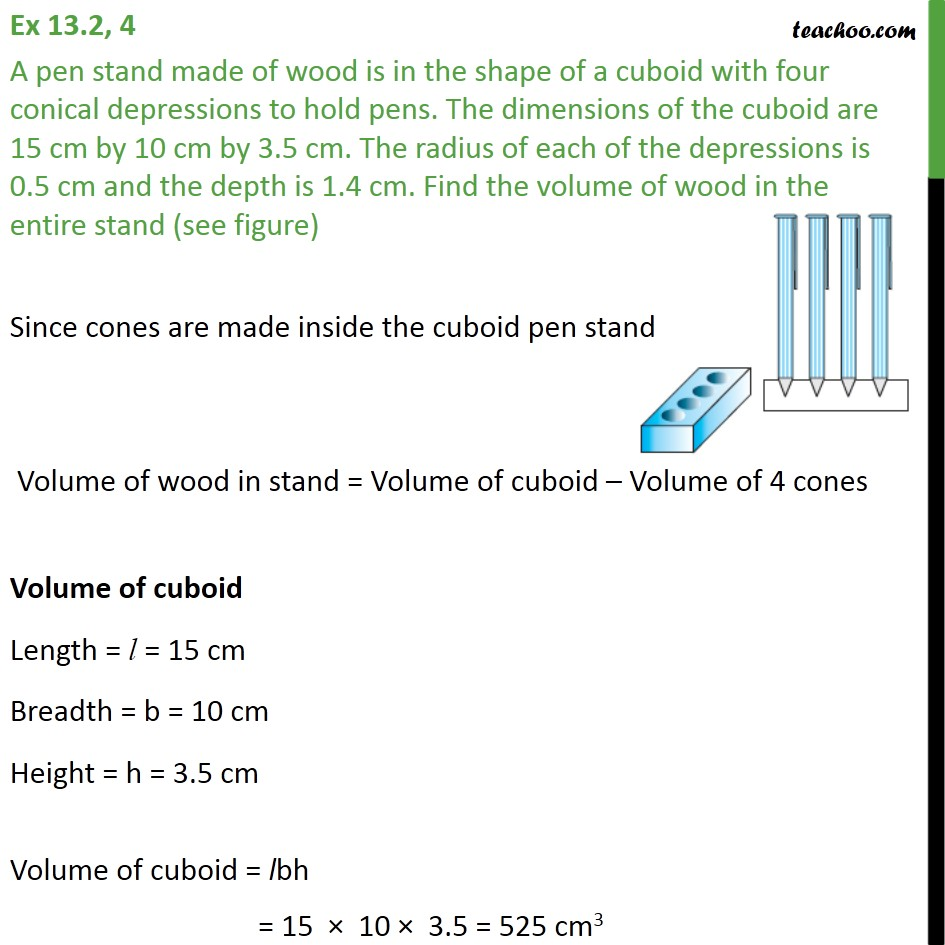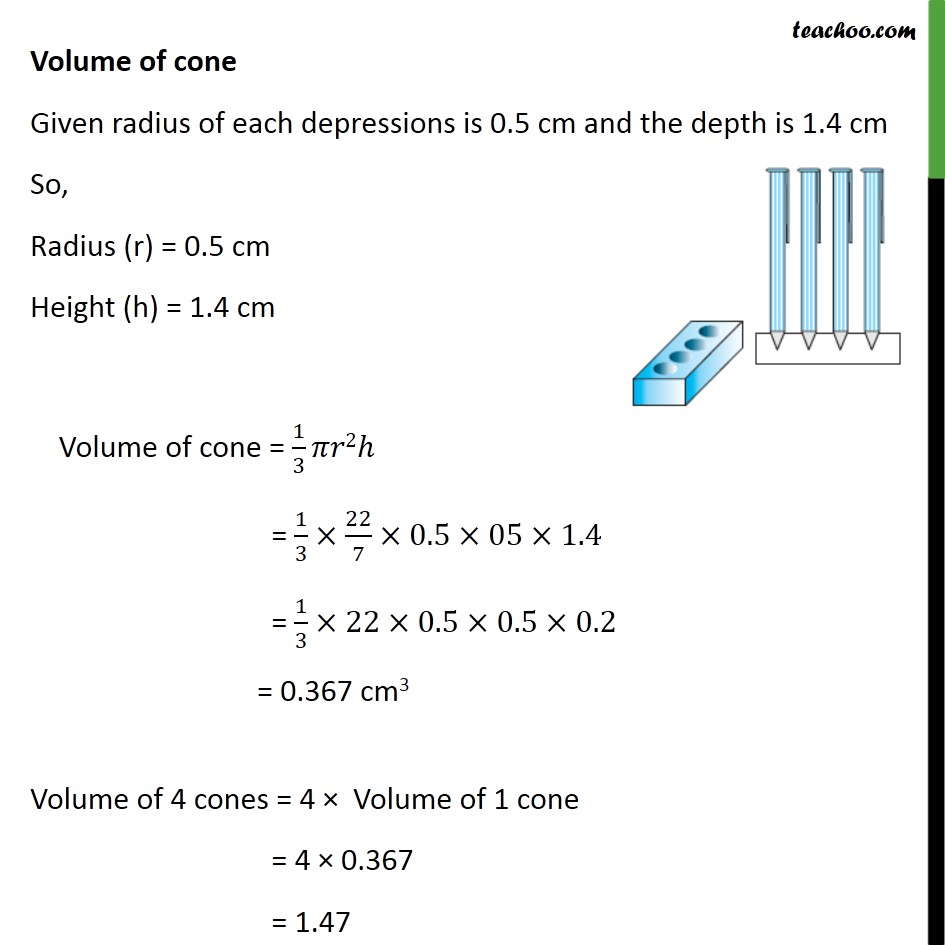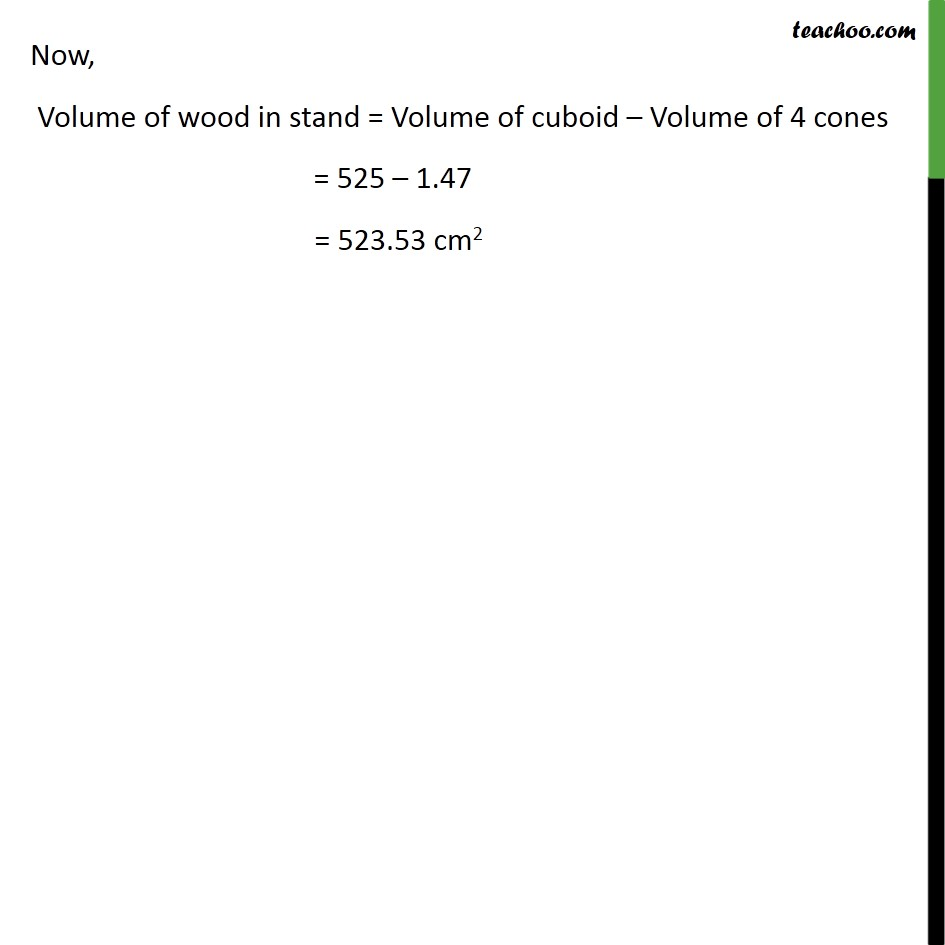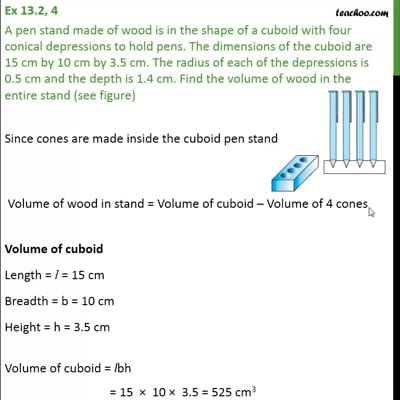Ex 13.2

Chapter 13 Class 10 Surface Areas and Volumes (Term 2)
Serial order wiseThis video is only available for Teachoo black users

### Transcript

Ex 13.2, 4 A pen stand made of wood is in the shape of a cuboid with four conical depressions to hold pens. The dimensions of the cuboid are 15 cm by 10 cm by 3.5 cm. The radius of each of the depressions is 0.5 cm and the depth is 1.4 cm. Find the volume of wood in the entire stand (see figure) Since cones are made inside the cuboid pen stand Volume of wood in stand = Volume of cuboid Volume of 4 cones Volume of cuboid Length = l = 15 cm Breadth = b = 10 cm Height = h = 3.5 cm Volume of cuboid = lbh = 15 10 3.5 = 525 cm3 Volume of cone Given radius of each depressions is 0.5 cm and the depth is 1.4 cm So, Radius (r) = 0.5 cm Height (h) = 1.4 cm Volume of cone = 1/3 2 = 1/3 22/7 0.5 05 1.4 = 1/3 22 0.5 0.5 0.2 = 0.367 cm3 Volume of 4 cones = 4 Volume of 1 cone = 4 0.367 = 1.47 Now, Volume of wood in stand = Volume of cuboid Volume of 4 cones = 525 1.47 = 523.53 cm2